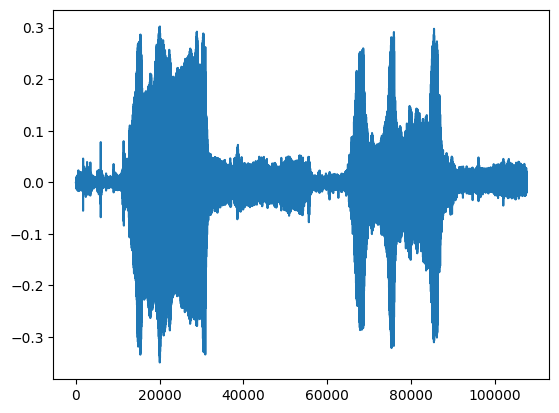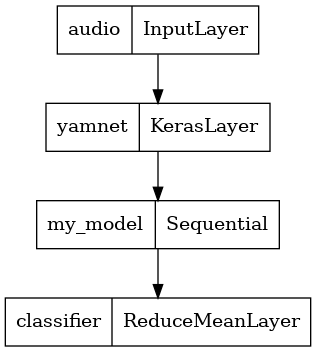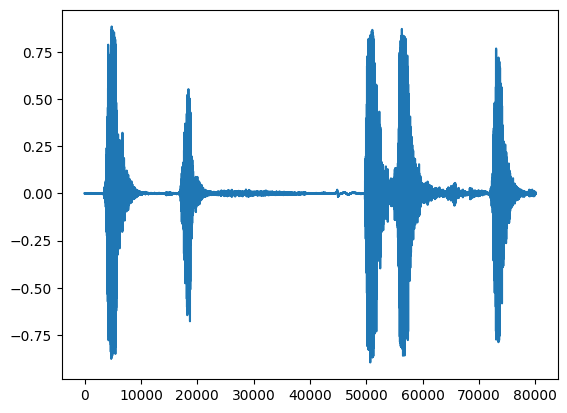# Transfer learning with YAMNet for environmental sound classification

YAMNet is a pre-trained deep neural network that can predict audio events from 521 classes, such as laughter, barking, or a siren.

In this tutorial you will learn how to:

• Load and use the YAMNet model for inference.
• Build a new model using the YAMNet embeddings to classify cat and dog sounds.
• Evaluate and export your model.

## Import TensorFlow and other libraries

Start by installing TensorFlow I/O, which will make it easier for you to load audio files off disk.

````pip install tensorflow_io`
```
``````import os

from IPython import display
import matplotlib.pyplot as plt
import numpy as np
import pandas as pd

import tensorflow as tf
import tensorflow_hub as hub
import tensorflow_io as tfio
``````

YAMNet is a pre-trained neural network that employs the MobileNetV1 depthwise-separable convolution architecture. It can use an audio waveform as input and make independent predictions for each of the 521 audio events from the AudioSet corpus.

Internally, the model extracts "frames" from the audio signal and processes batches of these frames. This version of the model uses frames that are 0.96 second long and extracts one frame every 0.48 seconds .

The model accepts a 1-D float32 Tensor or NumPy array containing a waveform of arbitrary length, represented as single-channel (mono) 16 kHz samples in the range `[-1.0, +1.0]`. This tutorial contains code to help you convert WAV files into the supported format.

The model returns 3 outputs, including the class scores, embeddings (which you will use for transfer learning), and the log mel spectrogram. You can find more details here.

One specific use of YAMNet is as a high-level feature extractor - the 1,024-dimensional embedding output. You will use the base (YAMNet) model's input features and feed them into your shallower model consisting of one hidden `tf.keras.layers.Dense` layer. Then, you will train the network on a small amount of data for audio classification without requiring a lot of labeled data and training end-to-end. (This is similar to transfer learning for image classification with TensorFlow Hub for more information.)

First, you will test the model and see the results of classifying audio. You will then construct the data pre-processing pipeline.

You are going to use a pre-trained YAMNet from Tensorflow Hub to extract the embeddings from the sound files.

Loading a model from TensorFlow Hub is straightforward: choose the model, copy its URL, and use the `load` function.

``````yamnet_model_handle = 'https://hub.tensorflow.google.cn/google/yamnet/1'
``````

``````testing_wav_file_name = tf.keras.utils.get_file('miaow_16k.wav',
cache_dir='./',
cache_subdir='test_data')

print(testing_wav_file_name)
``````
```Downloading data from https://storage.googleapis.com/audioset/miaow_16k.wav
221184/215546 [==============================] - 0s 0us/step
./test_data/miaow_16k.wav
```

You will need a function to load audio files, which will also be used later when working with the training data. (Learn more about reading audio files and their labels in Simple audio recognition.

``````# Utility functions for loading audio files and making sure the sample rate is correct.

@tf.function
""" Load a WAV file, convert it to a float tensor, resample to 16 kHz single-channel audio. """
wav, sample_rate = tf.audio.decode_wav(
file_contents,
desired_channels=1)
wav = tf.squeeze(wav, axis=-1)
sample_rate = tf.cast(sample_rate, dtype=tf.int64)
wav = tfio.audio.resample(wav, rate_in=sample_rate, rate_out=16000)
return wav
``````
``````testing_wav_data = load_wav_16k_mono(testing_wav_file_name)

_ = plt.plot(testing_wav_data)

# Play the audio file.
display.Audio(testing_wav_data,rate=16000)
``````
```WARNING:tensorflow:From /tmpfs/src/tf_docs_env/lib/python3.7/site-packages/tensorflow/python/ops/parallel_for/pfor.py:2382: calling gather (from tensorflow.python.ops.array_ops) with validate_indices is deprecated and will be removed in a future version.
Instructions for updating:
The `validate_indices` argument has no effect. Indices are always validated on CPU and never validated on GPU.
WARNING:tensorflow:From /tmpfs/src/tf_docs_env/lib/python3.7/site-packages/tensorflow/python/ops/parallel_for/pfor.py:2382: calling gather (from tensorflow.python.ops.array_ops) with validate_indices is deprecated and will be removed in a future version.
Instructions for updating:
The `validate_indices` argument has no effect. Indices are always validated on CPU and never validated on GPU.
WARNING:tensorflow:Using a while_loop for converting IO>AudioResample
WARNING:tensorflow:Using a while_loop for converting IO>AudioResample
```It's important to load the class names that YAMNet is able to recognize. The mapping file is present at `yamnet_model.class_map_path()` in the CSV format.

``````class_map_path = yamnet_model.class_map_path().numpy().decode('utf-8')

for name in class_names[:20]:
print(name)
print('...')
``````
```Speech
Child speech, kid speaking
Conversation
Narration, monologue
Babbling
Speech synthesizer
Shout
Bellow
Whoop
Yell
Children shouting
Screaming
Whispering
Laughter
Baby laughter
Giggle
Snicker
Belly laugh
Chuckle, chortle
Crying, sobbing
...
```

### Run inference

YAMNet provides frame-level class-scores (i.e., 521 scores for every frame). In order to determine clip-level predictions, the scores can be aggregated per-class across frames (e.g., using mean or max aggregation). This is done below by `scores_np.mean(axis=0)`. Finally, to find the top-scored class at the clip-level, you take the maximum of the 521 aggregated scores.

``````scores, embeddings, spectrogram = yamnet_model(testing_wav_data)
class_scores = tf.reduce_mean(scores, axis=0)
top_class = tf.argmax(class_scores)
inferred_class = class_names[top_class]

print(f'The main sound is: {inferred_class}')
print(f'The embeddings shape: {embeddings.shape}')
``````
```The main sound is: Animal
The embeddings shape: (13, 1024)
```

## ESC-50 dataset

The ESC-50 dataset (Piczak, 2015) is a labeled collection of 2,000 five-second long environmental audio recordings. The dataset consists of 50 classes, with 40 examples per class.

``````_ = tf.keras.utils.get_file('esc-50.zip',
'https://github.com/karoldvl/ESC-50/archive/master.zip',
cache_dir='./',
cache_subdir='datasets',
extract=True)
``````
```Downloading data from https://github.com/karoldvl/ESC-50/archive/master.zip
645701632/Unknown - 41s 0us/step
```

### Explore the data

The metadata for each file is specified in the csv file at `./datasets/ESC-50-master/meta/esc50.csv`

and all the audio files are in `./datasets/ESC-50-master/audio/`

You will create a pandas `DataFrame` with the mapping and use that to have a clearer view of the data.

``````esc50_csv = './datasets/ESC-50-master/meta/esc50.csv'
base_data_path = './datasets/ESC-50-master/audio/'

``````

### Filter the data

Now that the data is stored in the `DataFrame`, apply some transformations:

• Filter out rows and use only the selected classes - `dog` and `cat`. If you want to use any other classes, this is where you can choose them.
• Amend the filename to have the full path. This will make loading easier later.
• Change targets to be within a specific range. In this example, `dog` will remain at `0`, but `cat` will become `1` instead of its original value of `5`.
``````my_classes = ['dog', 'cat']
map_class_to_id = {'dog':0, 'cat':1}

filtered_pd = pd_data[pd_data.category.isin(my_classes)]

class_id = filtered_pd['category'].apply(lambda name: map_class_to_id[name])
filtered_pd = filtered_pd.assign(target=class_id)

full_path = filtered_pd['filename'].apply(lambda row: os.path.join(base_data_path, row))
filtered_pd = filtered_pd.assign(filename=full_path)

``````

### Load the audio files and retrieve embeddings

Here you'll apply the `load_wav_16k_mono` and prepare the WAV data for the model.

When extracting embeddings from the WAV data, you get an array of shape `(N, 1024)` where `N` is the number of frames that YAMNet found (one for every 0.48 seconds of audio).

Your model will use each frame as one input. Therefore, you need to create a new column that has one frame per row. You also need to expand the labels and the `fold` column to proper reflect these new rows.

The expanded `fold` column keeps the original values. You cannot mix frames because, when performing the splits, you might end up having parts of the same audio on different splits, which would make your validation and test steps less effective.

``````filenames = filtered_pd['filename']
targets = filtered_pd['target']
folds = filtered_pd['fold']

main_ds = tf.data.Dataset.from_tensor_slices((filenames, targets, folds))
main_ds.element_spec
``````
```(TensorSpec(shape=(), dtype=tf.string, name=None),
TensorSpec(shape=(), dtype=tf.int64, name=None),
TensorSpec(shape=(), dtype=tf.int64, name=None))
```
``````def load_wav_for_map(filename, label, fold):

main_ds.element_spec
``````
```WARNING:tensorflow:Using a while_loop for converting IO>AudioResample
WARNING:tensorflow:Using a while_loop for converting IO>AudioResample
(TensorSpec(shape=<unknown>, dtype=tf.float32, name=None),
TensorSpec(shape=(), dtype=tf.int64, name=None),
TensorSpec(shape=(), dtype=tf.int64, name=None))
```
``````# applies the embedding extraction model to a wav data
def extract_embedding(wav_data, label, fold):
''' run YAMNet to extract embedding from the wav data '''
scores, embeddings, spectrogram = yamnet_model(wav_data)
num_embeddings = tf.shape(embeddings)
return (embeddings,
tf.repeat(label, num_embeddings),
tf.repeat(fold, num_embeddings))

# extract embedding
main_ds = main_ds.map(extract_embedding).unbatch()
main_ds.element_spec
``````
```(TensorSpec(shape=(1024,), dtype=tf.float32, name=None),
TensorSpec(shape=(), dtype=tf.int64, name=None),
TensorSpec(shape=(), dtype=tf.int64, name=None))
```

### Split the data

You will use the `fold` column to split the dataset into train, validation and test sets.

ESC-50 is arranged into five uniformly-sized cross-validation `fold`s, such that clips from the same original source are always in the same `fold` - find out more in the ESC: Dataset for Environmental Sound Classification paper.

The last step is to remove the `fold` column from the dataset since you're not going to use it during training.

``````cached_ds = main_ds.cache()
train_ds = cached_ds.filter(lambda embedding, label, fold: fold < 4)
val_ds = cached_ds.filter(lambda embedding, label, fold: fold == 4)
test_ds = cached_ds.filter(lambda embedding, label, fold: fold == 5)

# remove the folds column now that it's not needed anymore
remove_fold_column = lambda embedding, label, fold: (embedding, label)

train_ds = train_ds.map(remove_fold_column)
val_ds = val_ds.map(remove_fold_column)
test_ds = test_ds.map(remove_fold_column)

train_ds = train_ds.cache().shuffle(1000).batch(32).prefetch(tf.data.AUTOTUNE)
val_ds = val_ds.cache().batch(32).prefetch(tf.data.AUTOTUNE)
test_ds = test_ds.cache().batch(32).prefetch(tf.data.AUTOTUNE)
``````

You did most of the work! Next, define a very simple Sequential model with one hidden layer and two outputs to recognize cats and dogs from sounds.

``````my_model = tf.keras.Sequential([
tf.keras.layers.Input(shape=(1024), dtype=tf.float32,
name='input_embedding'),
tf.keras.layers.Dense(512, activation='relu'),
tf.keras.layers.Dense(len(my_classes))
], name='my_model')

my_model.summary()
``````
```WARNING:tensorflow:Please add `keras.layers.InputLayer` instead of `keras.Input` to Sequential model. `keras.Input` is intended to be used by Functional model.
WARNING:tensorflow:Please add `keras.layers.InputLayer` instead of `keras.Input` to Sequential model. `keras.Input` is intended to be used by Functional model.
Model: "my_model"
_________________________________________________________________
Layer (type)                 Output Shape              Param #
=================================================================
dense (Dense)                (None, 512)               524800
_________________________________________________________________
dense_1 (Dense)              (None, 2)                 1026
=================================================================
Total params: 525,826
Trainable params: 525,826
Non-trainable params: 0
_________________________________________________________________
```
``````my_model.compile(loss=tf.keras.losses.SparseCategoricalCrossentropy(from_logits=True),
metrics=['accuracy'])

callback = tf.keras.callbacks.EarlyStopping(monitor='loss',
patience=3,
restore_best_weights=True)
``````
``````history = my_model.fit(train_ds,
epochs=20,
validation_data=val_ds,
callbacks=callback)
``````
```Epoch 1/20
15/15 [==============================] - 5s 25ms/step - loss: 0.7833 - accuracy: 0.8000 - val_loss: 0.6789 - val_accuracy: 0.8687
Epoch 2/20
15/15 [==============================] - 0s 16ms/step - loss: 0.5082 - accuracy: 0.8958 - val_loss: 0.3775 - val_accuracy: 0.8813
Epoch 3/20
15/15 [==============================] - 0s 17ms/step - loss: 0.3210 - accuracy: 0.8750 - val_loss: 0.5043 - val_accuracy: 0.8750
Epoch 4/20
15/15 [==============================] - 0s 17ms/step - loss: 0.2146 - accuracy: 0.9021 - val_loss: 0.3757 - val_accuracy: 0.8750
Epoch 5/20
15/15 [==============================] - 0s 17ms/step - loss: 0.2113 - accuracy: 0.9062 - val_loss: 0.2740 - val_accuracy: 0.8750
Epoch 6/20
15/15 [==============================] - 0s 17ms/step - loss: 0.2672 - accuracy: 0.9167 - val_loss: 0.4483 - val_accuracy: 0.8750
Epoch 7/20
15/15 [==============================] - 0s 17ms/step - loss: 0.2386 - accuracy: 0.9333 - val_loss: 0.5775 - val_accuracy: 0.8687
Epoch 8/20
15/15 [==============================] - 0s 17ms/step - loss: 0.1639 - accuracy: 0.9229 - val_loss: 0.4539 - val_accuracy: 0.8750
Epoch 9/20
15/15 [==============================] - 0s 18ms/step - loss: 0.3539 - accuracy: 0.9250 - val_loss: 0.2091 - val_accuracy: 0.9187
Epoch 10/20
15/15 [==============================] - 0s 18ms/step - loss: 0.2705 - accuracy: 0.9271 - val_loss: 0.2505 - val_accuracy: 0.9062
Epoch 11/20
15/15 [==============================] - 0s 17ms/step - loss: 0.2582 - accuracy: 0.9312 - val_loss: 0.2182 - val_accuracy: 0.9250
```

Let's run the `evaluate` method on the test data just to be sure there's no overfitting.

``````loss, accuracy = my_model.evaluate(test_ds)

print("Loss: ", loss)
print("Accuracy: ", accuracy)
``````
```5/5 [==============================] - 0s 4ms/step - loss: 0.6575 - accuracy: 0.8125
Loss:  0.657511293888092
Accuracy:  0.8125
```

You did it!

Next, try your model on the embedding from the previous test using YAMNet only.

``````scores, embeddings, spectrogram = yamnet_model(testing_wav_data)
result = my_model(embeddings).numpy()

inferred_class = my_classes[result.mean(axis=0).argmax()]
print(f'The main sound is: {inferred_class}')
``````
```The main sound is: cat
```

## Save a model that can directly take a WAV file as input

Your model works when you give it the embeddings as input.

In a real-world scenario, you'll want to use audio data as a direct input.

To do that, you will combine YAMNet with your model into a single model that you can export for other applications.

To make it easier to use the model's result, the final layer will be a `reduce_mean` operation. When using this model for serving (which you will learn about later in the tutorial), you will need the name of the final layer. If you don't define one, TensorFlow will auto-define an incremental one that makes it hard to test, as it will keep changing every time you train the model. When using a raw TensorFlow operation, you can't assign a name to it. To address this issue, you'll create a custom layer that applies `reduce_mean` and call it `'classifier'`.

``````class ReduceMeanLayer(tf.keras.layers.Layer):
def __init__(self, axis=0, **kwargs):
super(ReduceMeanLayer, self).__init__(**kwargs)
self.axis = axis

def call(self, input):
return tf.math.reduce_mean(input, axis=self.axis)
``````
``````saved_model_path = './dogs_and_cats_yamnet'

input_segment = tf.keras.layers.Input(shape=(), dtype=tf.float32, name='audio')
embedding_extraction_layer = hub.KerasLayer(yamnet_model_handle,
trainable=False, name='yamnet')
_, embeddings_output, _ = embedding_extraction_layer(input_segment)
serving_outputs = my_model(embeddings_output)
serving_outputs = ReduceMeanLayer(axis=0, name='classifier')(serving_outputs)
serving_model = tf.keras.Model(input_segment, serving_outputs)
serving_model.save(saved_model_path, include_optimizer=False)
``````
```WARNING:tensorflow:Compiled the loaded model, but the compiled metrics have yet to be built. `model.compile_metrics` will be empty until you train or evaluate the model.
WARNING:tensorflow:Compiled the loaded model, but the compiled metrics have yet to be built. `model.compile_metrics` will be empty until you train or evaluate the model.
INFO:tensorflow:Assets written to: ./dogs_and_cats_yamnet/assets
INFO:tensorflow:Assets written to: ./dogs_and_cats_yamnet/assets
```
``````tf.keras.utils.plot_model(serving_model)
````````````reloaded_model = tf.saved_model.load(saved_model_path)
``````

And for the final test: given some sound data, does your model return the correct result?

``````reloaded_results = reloaded_model(testing_wav_data)
print(f'The main sound is: {cat_or_dog}')
``````
```The main sound is: cat
```

If you want to try your new model on a serving setup, you can use the 'serving_default' signature.

``````serving_results = reloaded_model.signatures['serving_default'](testing_wav_data)
cat_or_dog = my_classes[tf.argmax(serving_results['classifier'])]
print(f'The main sound is: {cat_or_dog}')
``````
```The main sound is: cat
```

## (Optional) Some more testing

Let's compare it to YAMNet on the test dataset.

``````test_pd = filtered_pd.loc[filtered_pd['fold'] == 5]
row = test_pd.sample(1)
filename = row['filename'].item()
print(filename)
print(f'Waveform values: {waveform}')
_ = plt.plot(waveform)

display.Audio(waveform, rate=16000)
``````
```./datasets/ESC-50-master/audio/5-212454-A-0.wav
WARNING:tensorflow:Using a while_loop for converting IO>AudioResample
WARNING:tensorflow:Using a while_loop for converting IO>AudioResample
Waveform values: [-8.8849301e-09  2.6603255e-08 -1.1731625e-08 ... -1.3478296e-03
-1.0509168e-03 -9.1038318e-04]
`````````# Run the model, check the output.
scores, embeddings, spectrogram = yamnet_model(waveform)
class_scores = tf.reduce_mean(scores, axis=0)
top_class = tf.argmax(class_scores)
inferred_class = class_names[top_class]
top_score = class_scores[top_class]
print(f'[YAMNet] The main sound is: {inferred_class} ({top_score})')

your_inferred_class = my_classes[your_top_class]
your_top_score = class_probabilities[your_top_class]
print(f'[Your model] The main sound is: {your_inferred_class} ({your_top_score})')
``````
```[YAMNet] The main sound is: Animal (0.9570276141166687)
[Your model] The main sound is: dog (0.9999711513519287)
```

## Next steps

You have created a model that can classify sounds from dogs or cats. With the same idea and a different dataset you can try, for example, building an acoustic identifier of birds based on their singing.

Share your project with the TensorFlow team on social media!

[]
[]